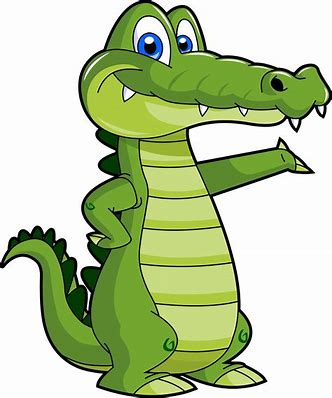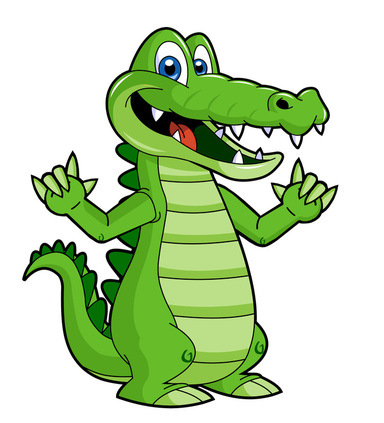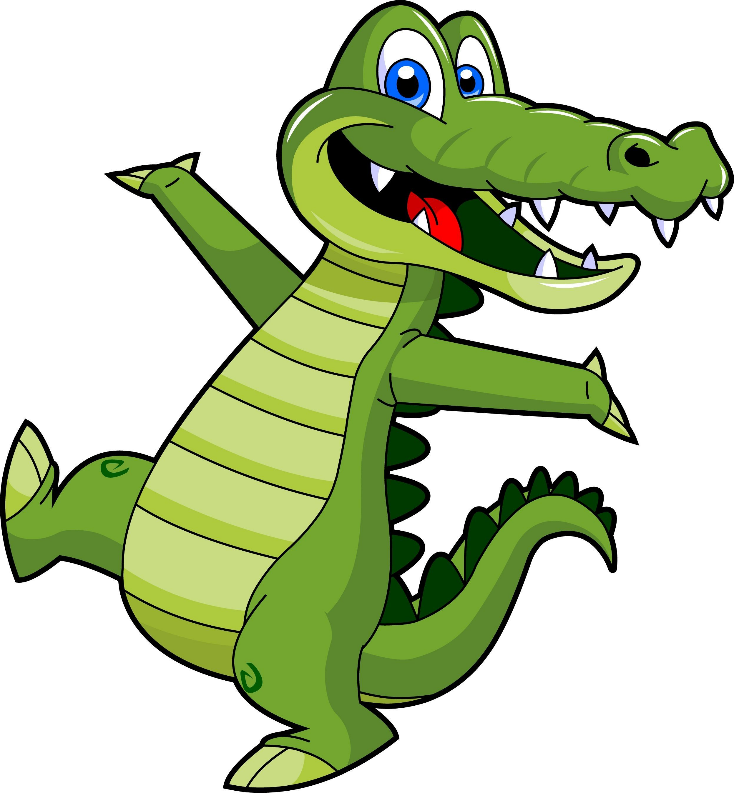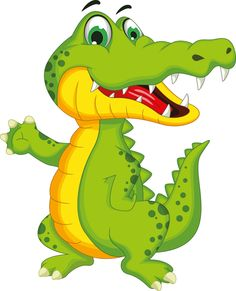# Alligator Math: Comparing 3 Digit-Numbers

Introduction

Today we will learn how to compare three-digit numbers based on their values.

Your friend Mr. Alligator is going to help us learn this lesson today! In the next pages, he will be sharing everything we will be going over today.In today’s lesson you will learn how to feed your friend Mr. Alligator!

You will first want to watch the YouTube videos, and this will show you how to feed Mr. Alligator!

Then you will complete a worksheet to show you mastered this lesson.Process
1. Check out the video below and it explains which symbol you use to compare the numbers.

1. Now since we know how to use these symbols, you will watch another video that will compare 2-digit numbers.

1. Now it is your turn to give it a try! Play the game that is listed below and show that you know how to compare numbers and feed your friend Mr. Alligator!

1. In class after reviewing all the attachments above, I will pass out a worksheet where you will compare 3-digit numbers and check your understandings.Evaluation

This is how you will be evaluated on your work.

 Beginning 1 Developing 2 Qualified 3 Exemplary 4 Score Can the students correctly identify the greater value? Student identified single digit numbers of greater value Student was able to identify a few greater values Student identified most of the greater values Student identified all greater values correctly Can the student correctly identify the lesser value? Student identified single digit numbers of lesser value Students identified a few lesser values Student identified most of the lesser values Student identified all lesser values correctly Did the student demonstrate understanding of the lesson? Student created a project but was unable to demonstrate understanding of the lesson Students created a project and demonstrated some understanding of the lesson with errors Student created a project and demonstrated understanding of the lesson with few errors Student accurately created a project demonstrating understanding of the lesson without any errors in the math Did the student accurately represent the equation? Student equation, number sentence, and structures were incorrect for the math problem they created Student equation, number sentence, and structures were partially correct for the math problem they created Student equation, number sentence, and structures were mostly correct for the math problem they created Student equation, number sentence, and structures demonstrate full understanding and correct representation of the math problem they created
Conclusion

I hope you guys enjoyed learning about the greater than, less than, or equal to symbols used when feeding Mr. Alligator!Credits